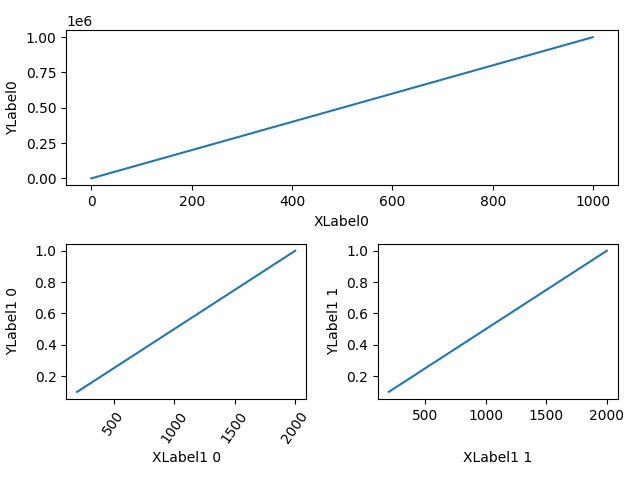# Aligning Labels¶

Aligning xlabel and ylabel using Figure.align_xlabels and Figure.align_ylabels

Figure.align_labels wraps these two functions.

Note that the xlabel "XLabel1 1" would normally be much closer to the x-axis, and "YLabel1 0" would be much closer to the y-axis of their respective axes.import matplotlib.pyplot as plt
import numpy as np
import matplotlib.gridspec as gridspec

fig = plt.figure(tight_layout=True)
gs = gridspec.GridSpec(2, 2)

ax.plot(np.arange(0, 1e6, 1000))
ax.set_ylabel('YLabel0')
ax.set_xlabel('XLabel0')

for i in range(2):